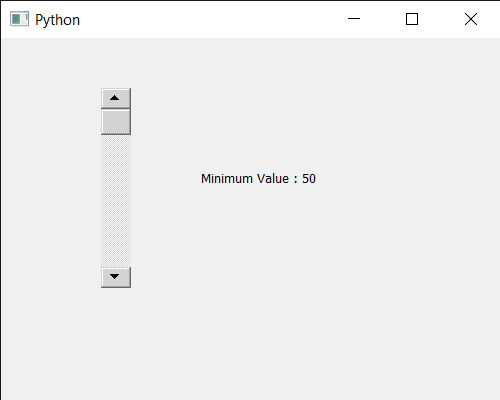Skip to content
Related Articles
PyQt5 QScrollBar – Getting Minimum Value
• Last Updated : 29 Jul, 2020

In this article we will see how we can get the minimum value of QScrollBar. QScrollBar is a control that enables the user to access parts of a document that is larger than the widget used to display it. Slider is the scroll-able object inside the bar. By default value range from 0 to 99. Value is basically depend upon the slider position, scroll bar value changes according to the position of the slider, value increases when slider goes down and decreases when slider moves up. It can be programmatically set with the help of `setValue` method. Minimum value is the value below which no value can be set to it, it can be set with the help of `setMinimum` method.

In order to do this we will use `minimum` method with the scroll bar object.

Syntax : scroll.minimum()

Argument : It takes no argument

Return : It returns integer

Below is the implementation

 `# importing libraries``from` `PyQt5.QtWidgets ``import` `*` `from` `PyQt5 ``import` `QtCore, QtGui``from` `PyQt5.QtGui ``import` `*` `from` `PyQt5.QtCore ``import` `*` `import` `sys`` ` ` ` `class` `Window(QMainWindow):`` ` `    ``def` `__init__(``self``):``        ``super``().__init__()`` ` `        ``# setting title``        ``self``.setWindowTitle(``"Python "``)`` ` `        ``# setting geometry``        ``self``.setGeometry(``100``, ``100``, ``500``, ``400``)`` ` `        ``# calling method``        ``self``.UiComponents()`` ` `        ``# showing all the widgets``        ``self``.show()`` ` ` ` ` ` `    ``# method for components``    ``def` `UiComponents(``self``):`` ` `        ``scroll ``=` `QScrollBar(``self``)`` ` `        ``# setting geometry of the scroll bar``        ``scroll.setGeometry(``100``, ``50``, ``30``, ``200``)`` ` `        ``# setting minimum value of scroll bar``        ``scroll.setMinimum(``50``)`` ` `        ``# making its backgorund color to green``        ``scroll.setStyleSheet(``"background : lightgrey;"``)`` ` `        ``# creating a label``        ``label ``=` `QLabel(``"GeesforGeeks"``, ``self``)`` ` `        ``# setting geometry to the label``        ``label.setGeometry(``200``, ``100``, ``300``, ``80``)`` ` `        ``# making label multi line``        ``label.setWordWrap(``True``)`` ` `        ``# getting value changed signal``        ``scroll.valueChanged.connect(``lambda``: do_action())`` ` `        ``# method called when signal is emitted``        ``def` `do_action():`` ` `            ``# getting current value``            ``value ``=` `scroll.value()`` ` `            ``# setting text to the label``            ``label.setText(``"Current Value : "` `+` `str``(value))`` ` ` ` `        ``# getting minimum value``        ``value ``=` `scroll.minimum()`` ` `        ``# setting text to the label``        ``label.setText(``"Minimum Value : "` `+` `str``(value))`` ` ` ` ` ` ` ` `# create pyqt5 app``App ``=` `QApplication(sys.argv)`` ` `# create the instance of our Window``window ``=` `Window()`` ` `# start the app``sys.exit(App.``exec``())`

Output :Attention geek! Strengthen your foundations with the Python Programming Foundation Course and learn the basics.

To begin with, your interview preparations Enhance your Data Structures concepts with the Python DS Course. And to begin with your Machine Learning Journey, join the Machine Learning – Basic Level Course

My Personal Notes arrow_drop_up